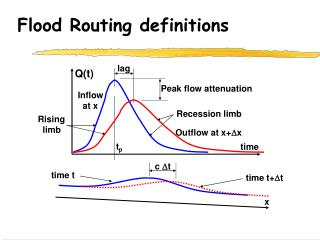DownloadDownload PresentationFlood Routing definitions

# Flood Routing definitions

Download Presentation## Flood Routing definitions

- - - - - - - - - - - - - - - - - - - - - - - - - - - E N D - - - - - - - - - - - - - - - - - - - - - - - - - - -
##### Presentation Transcript

1. lag Peak flow attenuation Recession limb Rising limb Outflow at x+Dx c Dt time t time t+Dt x Flood Routing definitions Q(t) Inflow at x tp time

2. Flood Routing methods • Hydraulic • Uses both dynamic and continuity equations • Allows backwater effects to be modelled • Solution advanced by timestep Dt • Hydrologic • Uses only continuity equation • Cannot model backwater effects • Solution advanced downstream by Dx

3. t+ t t A Q Q+Q x Kinematic Wave Equation Continuity with no lateral inflow yields: For quasi-uniform flow: Substitute and separate variables to get wave eq. or where c = dQ/dA is wave celerity

4. Space-Time Coordinates Time t a Dx Flow Q4 unknown 3 4 8 5 6 Nucleus Dt b Dt 7 1 2 Dx Distance x

5. 3 8 4 5 6 7 2 1 Continuity Around the Nucleus bdt adx

6. Generalized Muskingum equation Let and get Q4=f(Q1 , Q2 , Q3) Collecting terms, Setting b = 0.5 yields where

7. or Convert the Wave equation to a Diffusion equation Diffusion coefficient is related to channel conveyance Deriving the Diffusion equation Non-centered finite difference scheme creates a numerical error

8. Compare the two equations for the diffusion coeff. D f(a,b,D)=0 leads to multiple sets of (a,b) coordinates for any value of D. Determine weighting coefficients

9. Numerical Stability Criteria Condition for numerical stability is Unstable

10. From parts 1 & 2 or Limits for Dx and Dt For b = 0.5 and For very long channels, route hydrograph over multiple sub-reaches of length Dx=Length/N, N = 2,3,4...

11. From parts 1 & 2 or or From parts 2 & 3 Limits for Dx and Dt For b = 0.5 and For very long channels, route hydrograph over multiple sub-reaches of length Dx=Length/N, N=2,3,4... For very short channels, use routing time-step equal to sub-multiple of hydrology time step, dt=Dt/N, N=2,3,4...

12. MIDUSS 98 Route Command

13. Estimated values of weighting coefficients Details of last conduit design are displayed Changes to Dx or Dt reported for information User can change computed X or K values MIDUSS 98 Route Command

14. Results of Route command

15. Calculating celerity